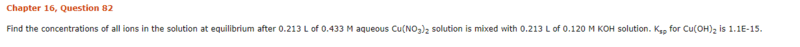# Concentration of Ions at Equilibrium

• Revengeance

## Homework Statement

Find the concentrations of all ions in the solution at equilibrium after 0.213 L of 0.433 M aqueous Cu(NO3)2 solution is mixed with 0.213 L of 0.120 M KOH solution. Ksp for Cu(OH)2 is 1.1E-15.

## Homework Equations

n=m/M

[Cu][OH]^2 = Ksp .. ?

## The Attempt at a Solution

ok so for the solution what i did was write out the balanced equation

Cu(NO3)2 + 2KOH -> Cu(OH)2 + 2K(NO3)

So we are told that the Cu(OH)2 = Ksp, so that means the spectator ions NO3 and K are going to be, their moles over the new volume. So i solved for them already and had received 0.061 mol/l K 0.433 mol NO3.

For Cu2+, what i did was i took the moles of Cu2+ initially, then i found moles KOH in terms of CuNO3, and i subtracted them, then divded the moles by the total volume to get 0.190 which is the same answer they got.

Now for OH, would i do the same process as above? Where i take the original moles of OH and then subtract it from moles of CuNO3 in terms of KOH, then divide that moles by the total volume?#### Attachments

Basically it is a limiting reagent problem first - check which ion (Cu2+ or OH-) is left in excess after the reaction. This will give you an easy to calculate concentration of this ion. Then use Ksp to calculate the concentration of the other ion.

Actually to be precise you should take into account both ions from the dissolution, but with so low Ksp it probably doesn't matter.

Basically it is a limiting reagent problem first - check which ion (Cu2+ or OH-) is left in excess after the reaction. This will give you an easy to calculate concentration of this ion. Then use Ksp to calculate the concentration of the other ion.

Actually to be precise you should take into account both ions from the dissolution, but with so low Ksp it probably doesn't matter.

Hmm... so how would i check if Cu2+ or OH- is the limiting reagent or left in excess? I already have the concentration of Cu2+ at equilibrium. So in order to calculate the OH, would i have to use Ksp = [OH]^2[Cu] ?

so how would i check if Cu2+ or OH- is the limiting reagent or left in excess?

Simple stoichiometry, google for limiting reagent

Your concentration of Cu2+ is almost OK, I got 0.187 M, no idea where the difference comes from, unless you rounded it down (but all the concentration data is given with 3SD, so this part of the answer should have 3 SD as well).

And yes, assuming you know the concentration of Cu2+ it is time to calculate OH- using Ksp.